# Focus

A type of arrangement of the trajectories of an autonomous system of first-order ordinary differential equations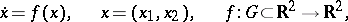(*), whereis the domain of uniqueness, in a neighbourhood of a singular point(cf. Equilibrium position). This type is characterized as follows. There is a neighbourhoodofsuch that for all trajectories of the system starting in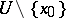, the negative semi-trajectories are escaping (in the course of time they leave any compact set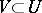) and the positive semi-trajectories, without leaving, tend to, winding round it like a logarithmic spiral, or conversely. The pointitself is also called a focus. The nature of the approach of the trajectories of the system tocan be described more precisely if one introduces polar coordinateson the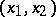-plane with pole at. Then for any semi-trajectory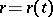,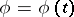,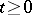(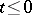), that tends to, the polar angle of the variable point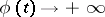(a left focus) or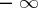(a right focus) as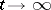.

A focus is either asymptotically Lyapunov stable or completely unstable (asymptotically stable as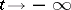). Its Poincaré index is 1. The figure depicts a right unstable focus at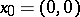.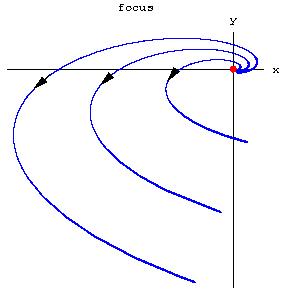Figure: f040700a

For a system (*) of class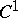() a singular pointis a focus in case the matrix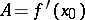has complex conjugate eigen values with non-zero real part, but it may also be a focus in case this matrix has purely imaginary or multiple real eigen values (see also Centre; Centre and focus problem).

For references see Singular point of a differential equation.